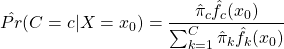## Resampling, the jackknife, and pseudo-observations

Resampling methods approximate the sampling distribution of a statistic or estimator. In essence, a sample taken from the population is treated as a population itself. A large number of new samples, or resamples, are taken from this “new population”, commonly with replacement, and within each of these resamples, the estimate of interest is re-obtained. A large number of these estimate replicates can then be used to construct the empirical sampling distribution from which confidence intervals, bias, and variance may be estimated. These methods are particularly advantageous for statistics or estimators for which no standard methods apply or are difficult to derive.

The jackknife is a popular resampling method, first introduced by Quenouille in 1949 as a method of bias estimation. In 1958, jackknifing was both named by Tukey and expanded to include variance estimation. A jackknife is a multipurpose tool, similar to a swiss army knife, that can get its user out of tricky situations. Efron later developed the arguably most popular resampling method, the bootstrap, in 1979 after being inspired by the jackknife.

Good simple ideas, of which the jackknife is a prime example, are our most precious intellectual commodity, so there is no need to apologize for the easy mathematical level.

Despite existing since the 1940’s, resampling methods were infeasible due to the computational power required to perform resampling and recalculate estimates many times. With today’s computing power, the uncomplicated yet powerful jackknife, and resampling methods more generally, should be a tool in every analyst’s toolbox.

## Parametric vs. Nonparametric Approach to Estimations

Parametric statistics assume that the unknown CDF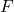belongs to a family of CDFs characterized by a parameter (vector)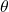. As the form ofis assumed, the target of estimation is its parameters. Thus, all uncertainty aboutis comprised of uncertainty about its parameters. Parameters are estimated by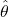, and estimates are be substituted into the assumed distribution to conduct inference for the quantities of interest. If the assumed distributionis incorrect, inference may also be inaccurate, or trends in the data may be missed.

To demonstrate the parametric approach, consider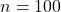independent and identically distributed random variables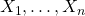generated from an exponential distribution with rate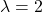. Investigators wish to estimate the 75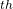percentile and erroneously assume that their data is normally distributed. Thus,is assumed to be the Normal CDF but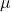and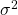are unknown. The parametersand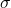are estimated in their typical way by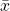and, respectively. Since the normal distribution belongs to the location-scale family, an estimate of the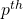percentile is provided by,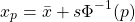where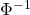is the standard normal quantile function, also known as the probit.

set.seed(12345)
library(tidyverse, quietly = T)

# Generate data from Exp(2)
x <- rexp(n = 100, rate = 2)

# True value of 75th percentile with rate = 2
true <- qexp(p = 0.75, rate = 2)
true

##  0.6931472

# Estimate mu and sigma
xbar <- mean(x)
s    <- sd(x)

# Estimate 75th percentile assuming mu = xbar and sigma = s
param_est <- xbar + s * qnorm(p = 0.75)
param_est

##  0.8792925


The true value of the 75percentile of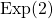is 0.69 while the parametric estimate is 0.88.

Nonparametric statistics make fewer distributions about the unknown distribution, requiring only mild assumptions such as continuity or the existence of specific moments. Instead of estimating parameters of,itself is the target of estimation.is commonly estimated by the empirical cumulative distribution function (ECDF)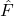,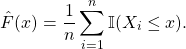Any statistic that can be expressed as a function of the CDF, known as a statistical functional and denoted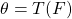, can be estimated by substitutingfor. That is, plug-in estimators can be obtained as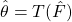.

## Motivation

For observed pairs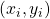,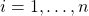, the relationship between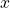and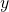can be defined generally as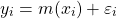where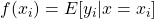and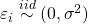. If we are unsure about the form of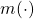, our objective may be to estimatewithout making too many assumptions about its shape. In other words, we aim to “let the data speak for itself”.

Simulated scatterplot of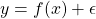. Here,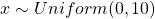and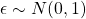. The true function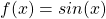is displayed in green.

Non-parametric approaches require only thatbe smooth and continuous. These assumptions are far less restrictive than alternative parametric approaches, thereby increasing the number of potential fits and providing additional flexibility. This makes non-parametric models particularly appealing when prior knowledge about‘s functional form is limited.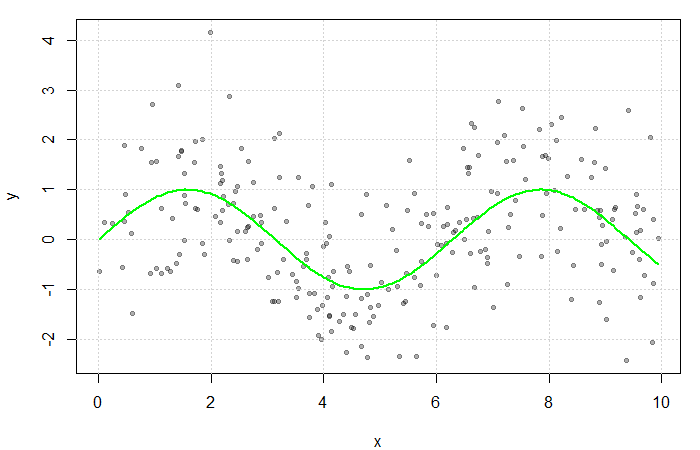## Estimating the Regression Function

If multiple values ofwere observed at each,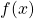could be estimated by averaging the value of the response at each. However, sinceis often continuous, it can take on a wide range of values making this quite rare. Instead, a neighbourhood ofis considered.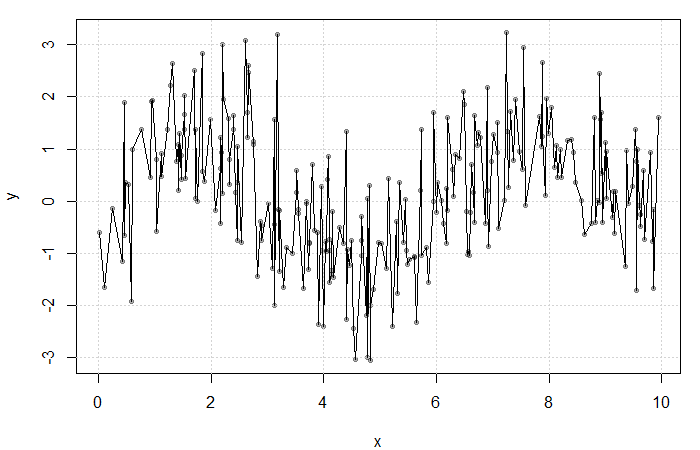Result of averaging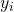at each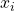. The fit is extremely rough due to gaps inand lowfrequency at each.

Define the neighbourhood aroundas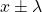for some bandwidth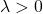. Then, a simple non-parametric estimate of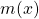can be constructed as average of the‘s corresponding to thewithin this neighbourhood. That is,

(1)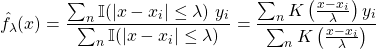where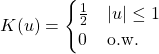is the uniform kernel. This estimator, referred to as the Nadaraya-Watson estimator, can be generalized to any kernel function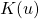(see my previous blog bost). It is, however, convention to use kernel functions of degree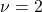(e.g. the Gaussian and Epanechnikov kernels).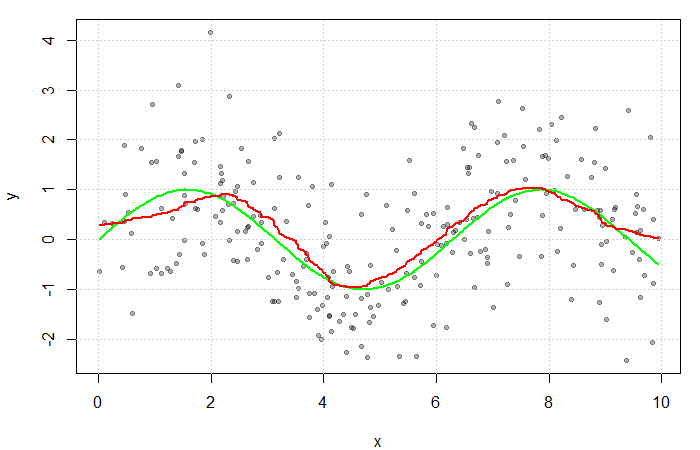The red line is the result of estimatingwith a Gaussian kernel and arbitrarily selected bandwidth of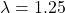. The green line represents the true function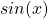.

## Motivation

It is important to have an understanding of some of the more traditional approaches to function estimation and classification before delving into the trendier topics of neural networks and decision trees. Many of these methods build on an understanding of each other and thus to truly be a MACHINE LEARNING MASTER, we’ve got to pay our dues. We will therefore start with the slightly less sexy topic of kernel density estimation.

Let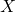be a random variable with a continuous distribution function (CDF)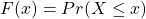and probability density function (PDF)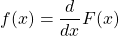Our goal is to estimatefrom a random sample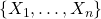. Estimation ofhas a number of applications including construction of the popular Naive Bayes classifier,